# Accounting Ratios Formulas and Ratio Analysis

There are various Accounting Ratios Formulas which are used by various stakeholders to evaluate and assess the business performance. For ease of reference, these are summarized as under : –

### CURRENT RATIO  AND LIQUIDITY  RATIO : –

Current Ratio =Liquid assets/Current liabilities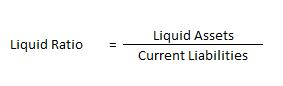### Explanation: –

Current Ratio Measures the ratio or proportion of Current Assets of a firm, to its current Liabilities . In terms of formula, it is written as under : –

Current ratio = Liquid assets/Current liabilities

### Solvency Ratio Analysis

Solvency Ratio Analysis : It measure the ability of a business to survive for a long period of time. These ratios are very important for stockholders and creditors as these ratios assess the ability of the firm to meet its long term liabilities.

The solvency ratios are categorised into following types :

a) Debt Equity Ratio

b) Total Assets to Debt Ratio

c) Proprietary Ratio

d) Interest Coverage Ratio

#### Debt Equity Ratio

It explains the relationship between long term debts and shareholder’s funds. The debt-equity ratio of 2:1 is considered as ideal.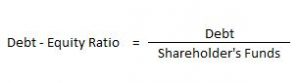Here, Debt includes Long-term Borrowings and Long-term Provisions. Shareholder’s Funds include Share Capital and Reserve & Surplus.

#### Total Assets to Debt Ratio

This ratio is a variation of debt-equity ratio. In this ratio, assets are expressed in terms of long term debts.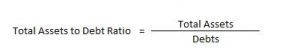Here, Total Assets = Non- Current Assets + Non-Current Investments + Long Term Loans & Advances + Current Assets

Debts = Long-term Borrowings and Long-term Provisions

#### Proprietary Ratio

It is the proportion of total assets funded by the shareholders. A higher proprietary ratio is an indicator of sound financial position from long-term point of view.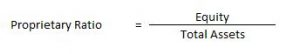#### Interest Coverage Ratio

The interest coverage ratio is a debt ratio and profitability ratio used to determine how easily a company can pay interest on its outstanding debt. This ratio indicates how many times the interest charges are covered by the profits available to pay interest charges.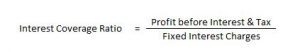### Activity Ratio Analysis

Activity Ratio Analysis – Activity ratios are financial analysis tools used to measure a business’ ability to convert its assets into cash. These ratios are known as turnover ratios because they indicate the rapidity with which the resources available to the concern are being used to produce revenue from operations.

The different types of activity ratios are:

1.  Inventory Turnover Ratio
4. Working Capital Turnover Ratio

#### Inventory Turnover Ratio

It indicates the relationship between the cost of revenue from operations during the year and average inventory during the year.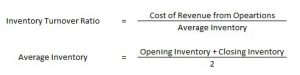Here, Cost of Revenue from Operations = Opening Inventory + Purchases + Carriage Inward + Wages + Other Direct Charges – Closing Inventory

It indicates the relationship between credit purchases and average trade payables during the year.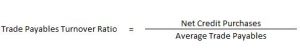Trade Payables include Creditors and Bills Payables.

It indicates the relationship between credit revenue from operations and average trade receivables during the year.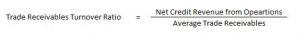#### Working Capital Turnover Ratio

This ratio is important in non-manufacturing concerns where current assets play a major role in generating sales. It indicates the efficient use of working capital.